Next: Suitable point for expansion Up: Various methods for algebraic Previous: Various methods for algebraic

## Series method

This method expands the expression

F(x,y, ... a,b,...) - G(x,y, ... m,n,... )

as a multivariate series in x, y, ... (all the main variables). If a match exists, all the coefficients must be equal to zero. So the matching problem is reduced to solving a system of equations in the parameter variables. Notice that each coefficient of the multivariate series will not contain any occurrence of the main variables, hence the coefficients are expressions in the parameters and the pattern variables. A solution of equating several coefficients to 0 in terms of the pattern variables should be substituted and checked in the original pattern. If at any point, a coefficient cannot be equated to zero, then there is no solution (provable) to the matching problem.

For example, to solve the matching of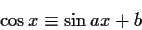we expand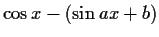around x=0 and obtain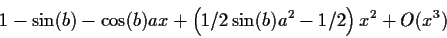The coefficient of the series must be equal to zero, this means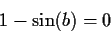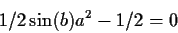From the first equation we conclude that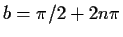for any integer n. This value of b satisfies the second equation. The third equation becomes a2-1=0 which has two solutions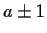. Plugging in any of these solutions in the original pattern, and simplifying, we arrive at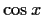and hence they are a solution of our matching problem.

This heuristic is very powerful, but it has several pitfalls. Some of them are avoidable.Next: Suitable point for expansion Up: Various methods for algebraic Previous: Various methods for algebraic
Gaston Gonnet
1999-07-04